Related Articles

# How to Calculate Weighted MAPE in Excel?

• Last Updated : 28 Jul, 2021

In statistics, we often use Forecasting Accuracy which denotes the closeness of a quantity to the actual value of that particular quantity. The actual value is also known as the true value. It basically denotes the degree of closeness or a verification process that is highly used by business professionals to keep track records of their sales and exchanges to maintain the demand and supply mapping every year. There are various methods to calculate Forecasting Accuracy.

So, one of the most common methods used to calculate the Forecasting Accuracy is MAPE which is abbreviated as Mean Absolute Percentage Error. It is an effective and more convenient method because it becomes easier to interpret the accuracy just by seeing the MAPE value. Here, we face a problem of infinite error when the Actual value of any entity becomes zero.

Attention reader! Don’t stop learning now. If you are an Excel beginner (or an intermediate) and want to learn Excel, Geeksforgeeks brings the perfect course for you to start, Diving Into Excel

WMAPE or Weighted MAPE abbreviated as Weighted Mean Absolute Percentage Error is also an accuracy prediction technique. Here, the problem of infinite error (divide by zero) is removed since the summation of actual value in the denominator can never be zero. It calculates the error based on weights but in case of MAPE error was calculated based on the average values. So, WMAPE is more reliable and gives efficient accuracy than MAPE.

The formula to calculate WMAPE in Excel is :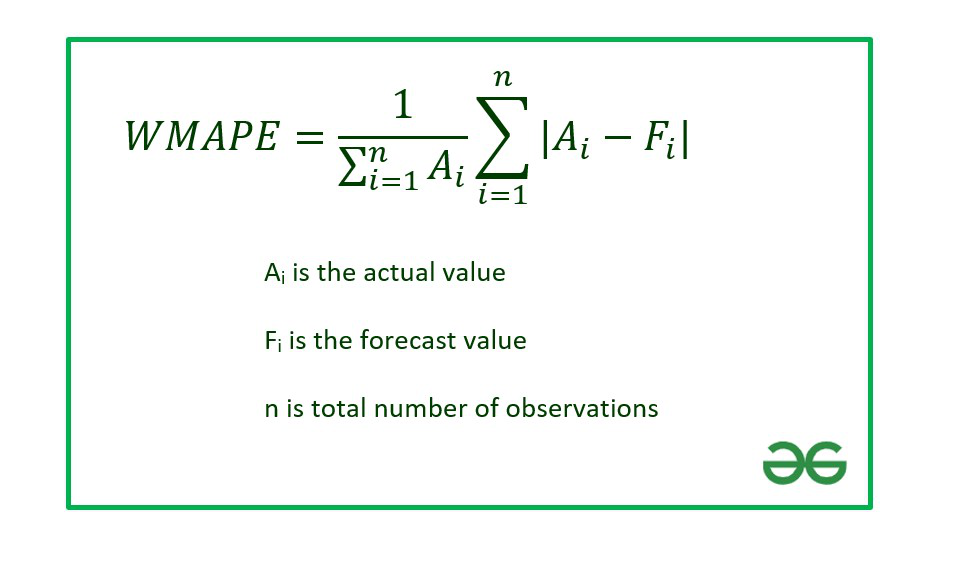In this article we are going to discuss how to calculate WMAPE in Excel using a suitable example.

Example : Consider the dataset shown below :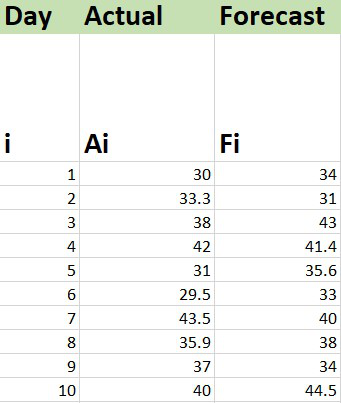## Calculation of WMAPE

The functions needed for formulas in Excel are-

SUM : To calculate the sum of multiple values

ABS : To calculate the absolute value.

The steps are :

1. Insert the data set in the Excel sheet.

2. Calculate the sub part of the formula inside the summation which is also known as Weighted Error.

```=ABS(Cell_No_Act-Cell_No_Fore)

where
ABS : Used to calculate the absolute value
Cell_No_Act : Cell number where Actual value is present
Cell_No_Fore : Cell number where Forecast value is present```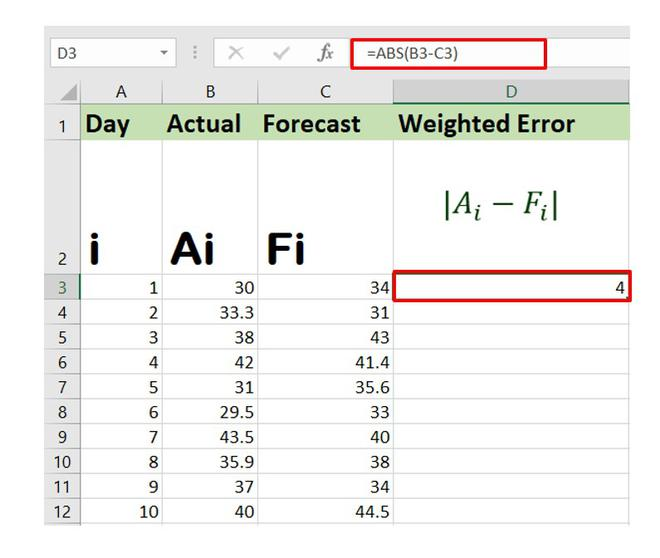The above formula will calculate the weighted error for the first entry in the data set. Now, you can drag the Auto Fill Options button to get the weighted error for the remaining entries.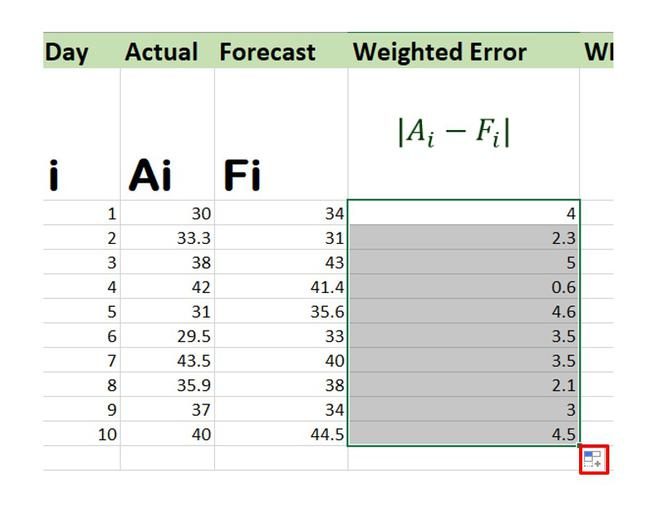3. Now use the SUM function to find the summation of both weighted errors and the actual values and divide them to get the WMAPE.

The entries of Weighted Error are in the Cell range : D3 to D12

The entries of Actual Value are in the Cell range : B3 to B12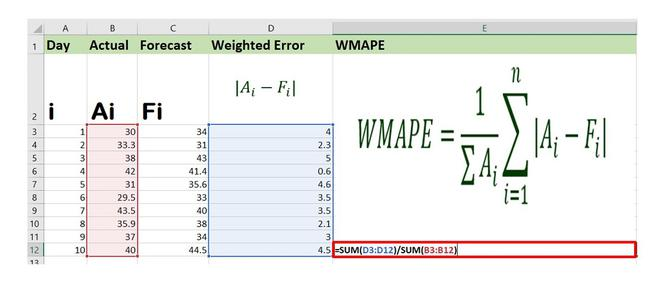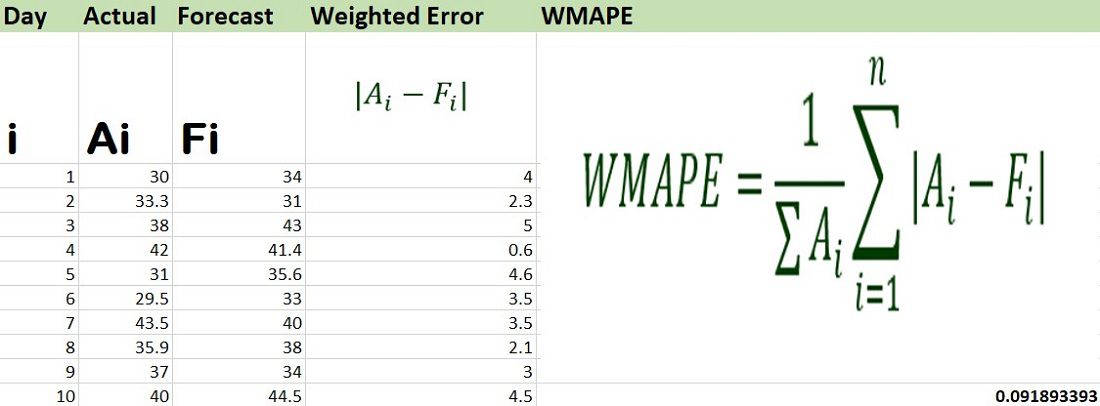The value of WMAPE is 0.09189 or 9.189% approximately.

My Personal Notes arrow_drop_up